# Capacitance

## DC Electric Circuits

• #### Question 1

Write an equation describing the precise mathematical relationship between electric charge (Q), capacitance (C), and voltage (V).

• #### Question 2

How does the rate of charge flow (current) into and out of a capacitor relate to the amount of voltage across its terminals? How does the rate of water flow into and out of a vessel relate to the amount of water stored in that vessel?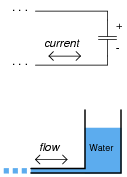• #### Question 3

One of the fundamental principles of calculus is a process called integration. This principle is important to understand because it is manifested in the behavior of capacitance. Thankfully, there are more familiar physical systems which also manifest the process of integration, making it easier to comprehend.

If we introduce a constant flow of water into a cylindrical tank with water, the water level inside that tank will rise at a constant rate over time: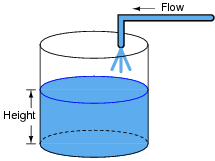In calculus terms, we would say that the tank integrates water flow into water height. That is, one quantity (flow) dictates the rate-of-change over time of another quantity (height).

Like the water tank, electrical capacitance also exhibits the phenomenon of integration with respect to time. Which electrical quantity (voltage or current) dictates the rate-of-change over time of which other quantity (voltage or current) in a capacitance? Or, to re-phrase the question, which quantity (voltage or current), when maintained at a constant value, results in which other quantity (current or voltage) steadily ramping either up or down over time?

• #### Question 4

Suppose two wires, separated by an air gap, are connected to opposite terminals on a voltage source (such as a battery). An electric field will develop in the space between the two wires: an invisible web of interaction, similar in some ways to a magnetic field. In this diagram, draw the invisible “lines of flux” for this electric field, showing their physical range:• #### Question 5

Electric fields may be described as “invisible webs” of interaction across space between electrically charged objects. Most people should be familiar with magnetic fields from playing with magnets as children: the forces of attraction or repulsion that act across open space between two or more magnetic objects. But electric fields are not the same as magnetic fields. The two different kinds of fields exert forces on entirely different objects.

Give an example of where an electric field manifests a tangible, physical force, like the magnetic fields we are all familiar with. Under what conditions are electric fields strong enough for human beings to detect without instruments?

• #### Question 6

Capacitance is a very important property in many types of electric circuits. Define what “capacitance” is, and what causes it.

• #### Question 7

The amount of capacitance between two conductors may be calculated by the following equation:

 C = εA d

Where,

ε = Permittivity of dielectric (absolute)

A = Conductor area, in square meters

d = Separation distance, in meters

How far away from each other would two metal plates, 2 square meters in area each, have to be in order to create a capacitance of 1 μF? Assume that the plates are separated by air.

• #### Question 8

Capacitance exists between any two conductors separated by an insulating medium. Given this fact, it makes sense that a length of two-conductor electrical cable will have capacitance distributed naturally along its length: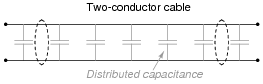There should be a way to prove the existence of such “stray” capacitance in a substantial length of two-conductor cable. Devise an experiment to do this.

• #### Question 9

Suppose you wished to build a component with no other purpose than to provide capacitance in an electric circuit (a capacitor). How might you design such a device to perform this function, and how could you maximize its capacitance?

• #### Question 10

What is a Leyden Jar, and how it its construction similar to the construction of all capacitors?

• #### Question 11

Electric fields, like all fields, have two fundamental measures: field force and field flux. In a capacitor, which of these field quantities is directly related to voltage between the plates, and which is directly related to the amount of charge (in coulombs) stored?

Based on this relationship, which electric field quantity changes when a sheet of glass is inserted between these two metal plates, connected to a source of constant voltage?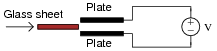• #### Question 12

The storage of electric charge in a capacitor is often likened to the storage of water in a vessel: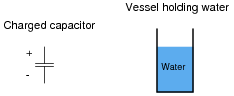Complete this analogy, relating the electrical quantities of charge (Q), voltage (E or V), and capacitance (C) to the quantities of water height, water volume, and vessel dimensions.

• #### Question 13

Suppose a mass is connected to a winch by means of a cable, and a person turns the winch drum to raise the mass off the ground: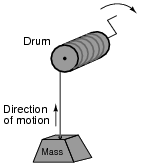A physicist would likely look at this scenario as an example of energy exchange: the person turning the drum is expending energy, which in turn is being stored in the mass in potential form.

Suppose now that the person stops turning the drum and instead engages a brake mechanism on the drum so that it reverses rotation and slowly allows the mass to return to ground level. Once again, a physicist would view this scenario as an exchange of energy: the mass is now releasing energy, while the brake mechanism is converting that released energy into heat: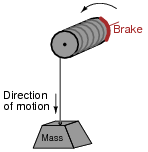In each of the above scenarios, draw arrows depicting directions of two forces: the force that the mass exerts on the drum, and the force that the drum exerts on the mass. Compare these force directions with the direction of motion in each scenario, and explain how these directions relate to the mass and drum alternately acting as energy source and energy load.

• #### Question 14

Draw the direction of current in this circuit, and also identify the polarity of the voltage across the battery and across the resistor. Then, compare the battery’s polarity with the direction of current through it, and the resistor’s polarity with the direction of current through it.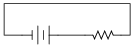What do you notice about the relationship between voltage polarity and current direction for these two different types of components? Identify the fundamental distinction between these two components that causes them to behave differently.

• #### Question 15

Suppose a capacitor is connected directly to an adjustable-voltage source, and the voltage of that source is steadily increased over time. We know that an increasing voltage across a capacitor will produce an electric field of increasing strength. Does this increase in electric field constitute an accumulation of energy in the capacitor, or a release of energy from the capacitor? In this scenario, does the capacitor act as a load or as a source of electrical energy?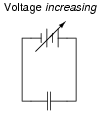Now, suppose the adjustable voltage source is steadily decreased over time. We know this will result in an electric field of decreasing strength in the capacitor. Does this decrease in electric field constitute an accumulation of energy in the capacitor, or a release of energy from the capacitor? In this scenario, does the capacitor act as a load or as a source of electrical energy?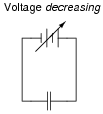For each of these scenarios, label the direction of current in the circuit.

• #### Question 16

Ohm’s Law tells us that the amount of current through a fixed resistance may be calculated as such:

 I = E R

We could also express this relationship in terms of conductance rather than resistance, knowing that G = 1/R:

 I = EG

However, the relationship between current and voltage for a fixed capacitance is quite different. The “Ohm’s Law” formula for a capacitor is as such:

 i = C de dt

What significance is there in the use of lower-case variables for current (i) and voltage (e)? Also, what does the expression de/dt mean? Note: in case you think that the d’s are variables, and should cancel out in this fraction, think again: this is no ordinary quotient! The d letters represent a calculus concept known as a differential, and a quotient of two d terms is called a derivative.

• #### Question 17

Complete this statement by substituting the correct electrical variables (voltage, current, resistance, capacitance):

Capacitors oppose changes in (fill-in-the-blank), reacting to such changes by producing a (fill-in-the-blank).
• #### Question 18

Electrical capacitance has a close mechanical analogy: elasticity. Explain what the term “elasticity” means for a mechanical spring, and how the quantities of velocity and force applied to a spring are respectively analogous to current and voltage applied to a capacitance.

• #### Question 19

Capacitors store energy in the form of an electric field. We may calculate the energy stored in a capacitance by integrating the product of capacitor voltage and capacitor current (P = IV) over time, since we know that power is the rate at which work (W) is done, and the amount of work done to a capacitor taking it from zero voltage to some non-zero amount of voltage constitutes energy stored (U):

 P = dW dt

 dW = P dt

 U = W = ⌠ ⌡ P dt

Find a way to substitute capacitance (C) and voltage (V) into the integrand so you may integrate to find an equation describing the amount of energy stored in a capacitor for any given capacitance and voltage values.